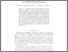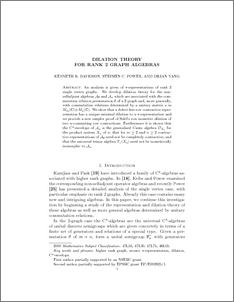# Dilation theory for rank two graph algebras.

Davidson, Kenneth R. and Power, Stephen C. and Yang, Dilian (2010) Dilation theory for rank two graph algebras. Journal of Operator Theory, 63 (2). pp. 245-270.Preview
PDF (DPYdilnMay28.pdf)
DPYdilnMay28.pdf

An analysis is given of $*$-representations of rank 2 single vertex graphs. We develop dilation theory for the non-selfadjoint algebras $\A_\theta$ and $\A_u$ which are associated with the commutation relation permutation $\theta$ of a 2-graph and, more generally, with commutation relations determined by a unitary matrix $u$ in $M_m(\bC) \otimes M_n(\bC)$. We show that a defect free row contractive representation has a unique minimal dilation to a $*$-representation and we provide a new simpler proof of Solel's row isometric dilation of two $u$-commuting row contractions. Furthermore it is shown that the $C^*$-envelope of $\A_u$ is the generalised Cuntz algebra $\O_{X_u}$ for the product system $X_u$ of $u$; that for $m\geqslant 2$ and $n \geqslant 2$ contractive representations of $\Ath$ need not be completely contractive; and that the universal tensor algebra $\T_+(X_u)$ need not be isometrically isomorphic to $\A_u$.View Item